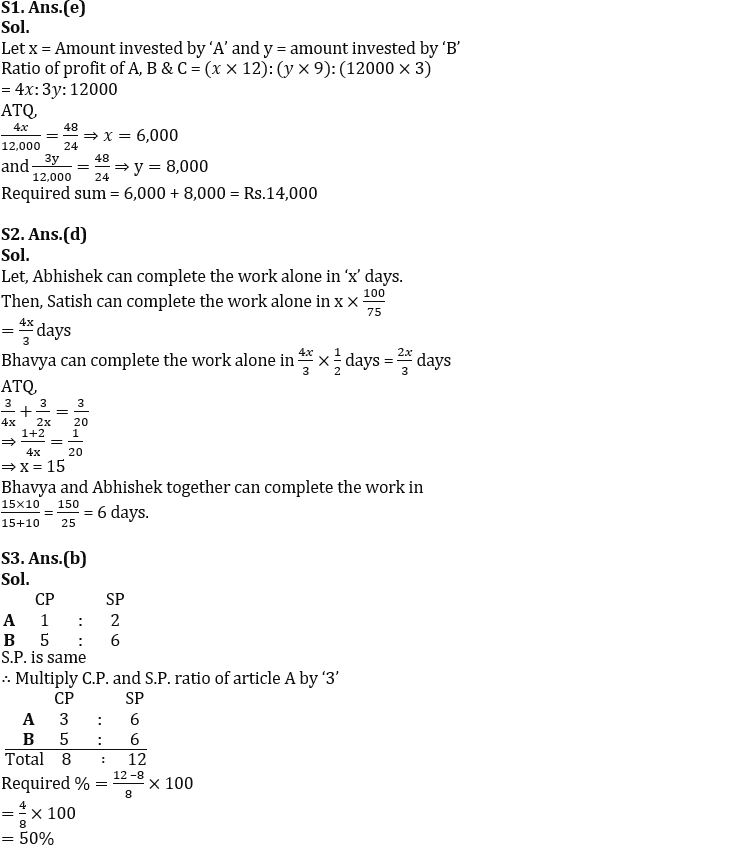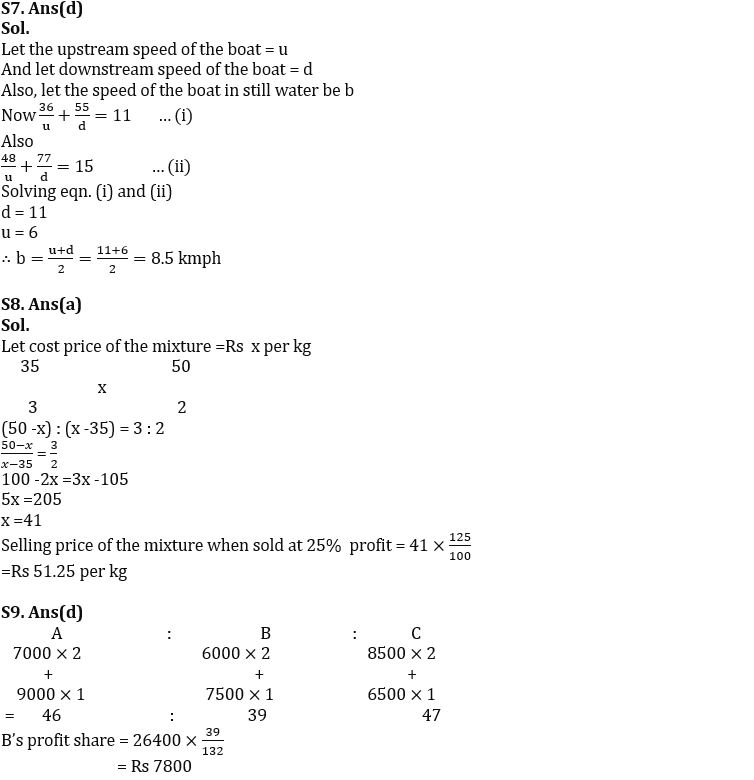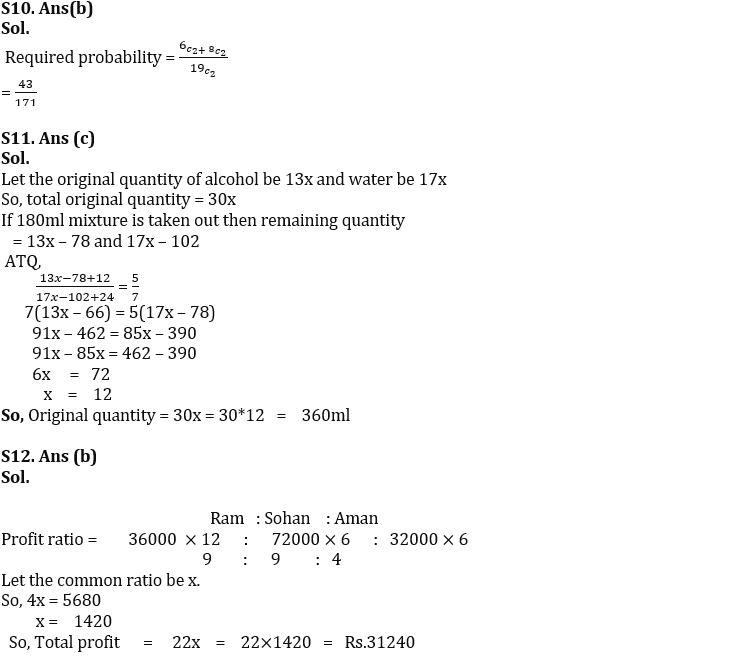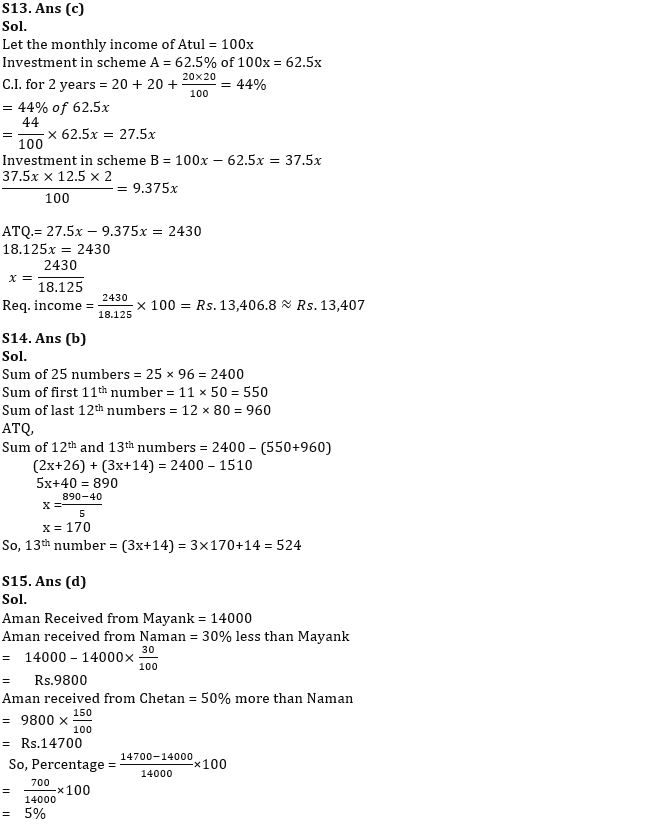Latest Banking jobs   »

# Quantitative Aptitude Quiz For IBPS Clerk/PO Prelims 2022- 5th September

Q1. A and B started a business with some amount. After 9 months B left the business & C joins the business with Rs. 12,000 and remains in business till the end of year. At the end of the year, profit share of A, B and C is Rs. 48, Rs. 48 and Rs. 24 respectively. Find the sum of the amount (in Rs.) invested by A and B together in the business?
(a) 8,000
(b) 10,000
(c) 15,000
(d) 12,000
(e) 14,000

Q2. Satish is 25% less efficient than Abhishek. Bhavya is 100% more efficient than Satish. If Satish, Bhavya together can complete the work in 20/3 days, find in how many days Bhavya & Abhishek together can complete the same work.
(a) 5 days
(b) 20/3 days
(c) 4 days
(d) 6 days
(e) 17/3 days

Q3. Man sold article A at 100% profit and article B at 20% profit. If selling price of article A and article B is same then find his overall profit percentage
(a) 40%
(b) 50%
(c) 55%
(d) 60%
(e) 75%

Q4. ⅔rd of first number is equal to the cube of the second number. If the second number is equal to 12% of 100, what is sum of the first & 2nd number?
(a) 2408
(b) 2640
(c) 2426
(d) 2604
(e) 2804

Q5. The present age of Bhagat and Abhi are in ratio of 9 : 8 respectively. After 10 years the ratio of their ages will be 10 : 9. What is the difference in their present age ?
(a) 8 years
(b) 6 years
(c) 12 years
(d) 4 years
(e) 10 years

Q6.Aakash invested Rs 16800 on simple interest at r % p.a. for 3 yrs and received Rs 7560 as total interest. Find the interest amount received by Aakash if the same amount is invested on compound interest at (r+5)% rate of interest after 2 yrs?
(a) Rs 7560
(b) Rs 7392
(c) Rs 7120
(d) Rs 7820
(e) Rs 7460

Q7.A boat can cover 36 km upstream and 55 km downstream in 11 hrs and 48 km upstream and 77 km downstream in 15 hrs, then find the speed of the boat in still water?
(a) 8 km/hr
(b) 6.5 km/hr
(c) 7.5 km/hr
(d) 8.5 km/hr
(e) 9 km/hr

Q8.Type A milk of cost price Rs 35 per litre is mixed with Type B milk having cost price rs 50 per litre in the ratio 3:2 respectively, then find the selling price(per kg) of the final mixture when sold at 25% profit ?(in rs)
(a) 51.25
(b) 48.75
(c) 53.3
(d) 57.4
(e) 53.5

Q9.A, B and C invested Rs 7000, Rs 6000 and Rs 8500 respectively in a partnership for 2 yrs .After 2 yrs, A and B increased their investment by Rs 2000 and Rs 1500 respectively and C decreased his investment by Rs 2000. At the end of three yrs, total profit received by them is Rs 26400, then find B’s profit share?
(a) Rs 8400
(b) Rs 9200
(c) Rs 7200
(d) Rs 7800
(e) Rs 8000

Q10.A bag contains 6 Blue balls, 5 Red balls and 8 yellow balls. If 2 balls are taken out from the bag randomly, then find the probability of both balls being of blue colour or yellow colour?
(a) 41/171
(b) 43/171
(c) 37/169
(d) 43/169
(e) 47/143

Q11. A mixture contains Alcohol and Water in the ratio of 13:17 respectively. If 180ml of mixture is taken out, and then 12ml of alcohol and 24ml of water is added into the remaining mixtures such that the ratio of alcohol and water becomes 5:7 respectively. Find the original quantity of the mixture.
(a) 345ml
(b) 480ml
(c) 360ml
(d) 520ml
(e) 640ml

Q12. Ram and Sohan entered into business investing Rs. 36000 and 72000 respectively. After 6 months, Sohan left and Aman entered into the business investing Rs. 32000. If at the end of the year, profit share of Aman was Rs. 5680 then find the total profit earned by all of them together.
(a) Rs. 17640
(b) Rs.31240
(c) Rs. 25795
(d) Rs. 16975
(e) Rs. 40460

Q13. Atul invested 62.5% of his monthly income in scheme A offering 20% C.I. (compounded annually) for 2 years and rest amount in scheme B offering 12.5% per annum simple interest for same period. If the difference between CI and SI is Rs. 2430 after 2 years, then find the monthly income(approx.) of Atul.
(a) Rs 15067
(b) Rs 16550
(c) Rs 13407
(d) Rs 12000
(e) Rs 18750

Q14. Average of 25 numbers is 96. If average of first 11 numbers is 50 while average of last 12 numbers is 80 and 12th and 13th numbers are (2x+26) and (3x+14) respectively. Find the 13th number.
(a) 624
(b) 524
(c) 575
(d) 325
(e) 765

Q15. Aman’s friend Mayank gave him Rs. 14000 and Naman gave to Aman 30% less than what Mayank gave to Aman. If Chetan gave 50% more than Naman to Aman, then the amount received by Aman from Chetan is what percent more than that of amount which is received by Aman from Mayank?
(a) 12.5%
(b) 2%
(c) 3%
(d) 5%
(e) 7.5%

Solutions#### Congratulations!Download Hindu Review of October 2021: Free PDF